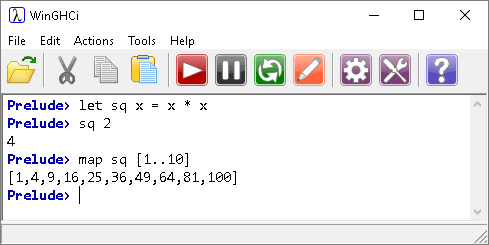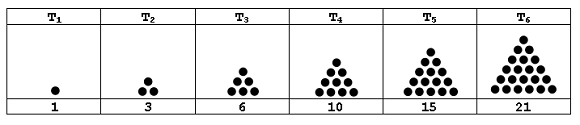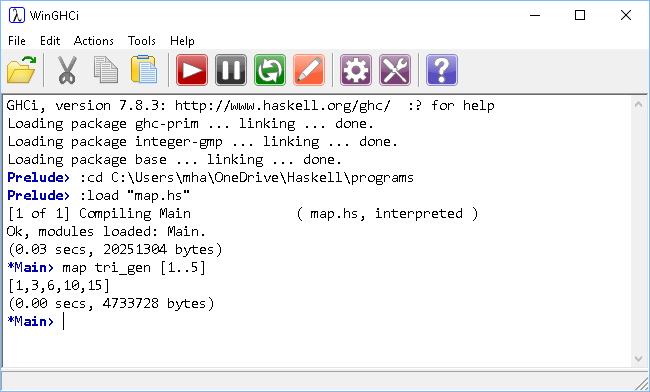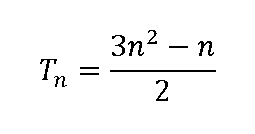## Introduction

The map function is a higher-order function that applies a given function to each element of a list, returning a list of results.

From the specification,

A function is higher-order if it takes a function as an argument or returns a function as a result, or does both.

The order of play for this function is,

map function list

In the example, a function is defined to return the square of a number.

The map function applies the sq function to each element in the list. A list of results is returned.Remember that operators are also functions in Haskell. With standard operators, you can achieve the same effect with,## Example

Triangular numbers are in the following form,One way to calculate the nth triangular number is the sum of the integers from 1 to n. We can write a simple function to do that and use it as an argument for the map function.

```triangular n = sum [1..n]
triangular_sequence = map triangular [1..10]
```Another way to calculate the nth triangular number, avoiding the summing, is with the following formula,This can be written as a function, like this,

```tri_gen x = floor (x*(x+1)/2)
```Pentagonal numbers can be generated using the following formula,You could write your own function to generate these and then map it onto a list.